﻿ EOF-LSTM神经网络的电离层TEC预报模型文章快速检索 高级检索
 大地测量与地球动力学2021, Vol. 41Issue (9): 911-915, 944  DOI: 10.14075/j.jgg.2021.09.005### 引用本文TANG Jun, LI Yinjian, ZHONG Zhengyu, et al. Prediction Model of Ionospheric TEC by EOF and LSTM Neural Network[J]. Journal of Geodesy and Geodynamics, 2021, 41(9): 911-915, 944.### Foundation support

National Natural Science Foundation of China, No. 41761089; Scientific Research Project of the Education Department of Jiangxi Province, No. GJJ190345; Special Innovation Fund Designated for Graduate Students of Jiangxi Province, No. YC2020-S358.

### 第一作者简介

TANG Jun, PhD, associate professor, majors in GNSS data processing and ionosphere model estimation, E-mail: tj928@163.com.

### 文章历史

EOF-LSTM神经网络的电离层TEC预报模型

1. 华东交通大学土木建筑学院，南昌市双港东大街808号，330013;
2. 华东交通大学土木工程国家实验教学示范中心，南昌市双港东大街808号，330013

1 模型原理 1.1 EOF分解

EOF分解是一种分析矩阵数据结构特征并提取主要信息的方法，在因子场统计组合、动力模型参数化、因子时间序列分析中已得到广泛应用，在统计预报领域往往作为统计组合的基础。EOF分解可以将场的时间序列分解为不随时间变化的空间特征和随时间变化的时间系数，继而通过方差贡献率剔除冗余信息，提取集中在若干个模态分量的主要信息。主要步骤为：

1) 设变量场Xm个测站的n组观测数据组成，可表示为矩阵形式：

 $\boldsymbol{X}_{m \times n}=\left[\begin{array}{cccc} x_{11} & x_{12} & \cdots & x_{1 n} \\ x_{21} & x_{22} & \cdots & x_{21} \\ \vdots & \vdots & \ddots & \vdots \\ x_{m 1} & x_{m 2} & \cdots & x_{m n} \end{array}\right]$ (1)

2) 将X转置并计算交叉积得到：

 $\boldsymbol{C}_{m \times m}=\frac{1}{n} \boldsymbol{X} \times \boldsymbol{X}^{\mathrm{T}}$ (2)

3) 计算方阵C的特征值λ(λ1, λ2, …, λm)和特征向量V，将特征值λ按大小顺序排列，即λ1>λ2 > … > λm，此时每个非0的特征值即代表对应的空间特征向量，称为EOF。将空间特征向量投影到原始资料上，得到时间系数Y

 $\boldsymbol{Y}=\boldsymbol{V}^{\mathrm{T}} \times \boldsymbol{X}$ (3)

4) 利用各时间系数的方差贡献率剔除冗余信息，计算公式为：

 $P_{i}=\lambda_{i} / \sum\limits_{i=1}^{m} \lambda_{i}$ (4)
1.2 EOF-LSTM预报模型

LSTM神经网络是一种特殊的循环神经网络(recurrent neural network，RNN)，其在RNN的基础上增加了隐藏神经元，解决了长序列训练过程中的梯度消失和梯度爆炸的问题，其内部结构如图 1所示。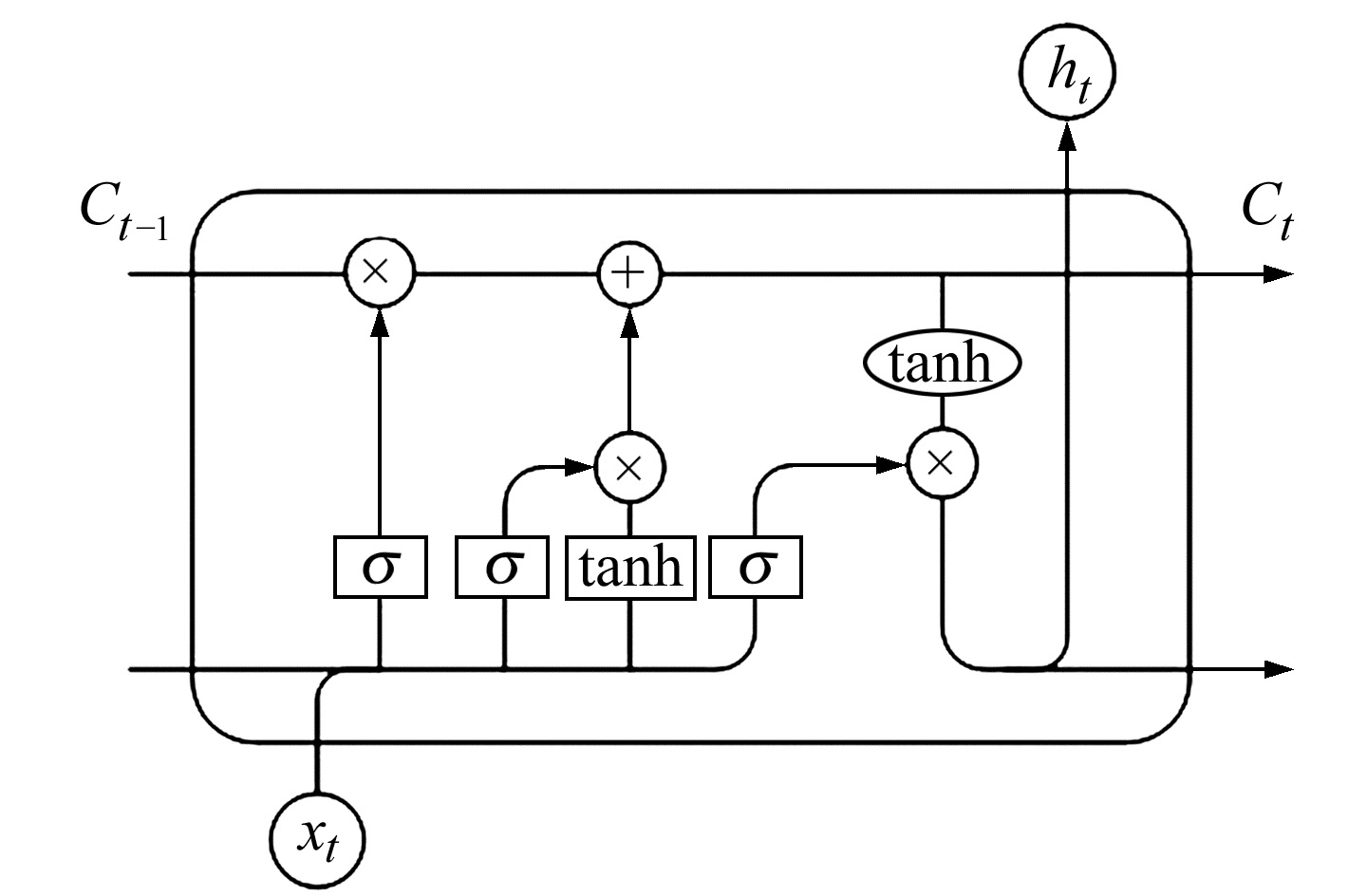图 1 LSTM神经网络内部结构 Fig. 1 Internal structure of LSTM neural network

LSTM神经网络单元通过遗忘门、输入门和输出门共同决定数据信息的流动，其中遗忘门的作用是筛选冗余信息，即将xtht压缩为0~1之间的数字以表示“遗忘”信息的程度，0表示“遗忘”所有信息，1表示保留所有信息。具体计算公式为：

 $f_{t}=\sigma\left(\boldsymbol{w}_{f} \cdot\left[h_{t-1}, x_{t}\right]+b_{f}\right)$ (5)

 $i_{t}=\sigma\left(\boldsymbol{w}_{i} \cdot\left[h_{t-1}, x_{t}\right]+b_{i}\right)$ (6)
 $\widetilde{C}_{t}=\tanh \left(\boldsymbol{w}_{C} \cdot\left[h_{t-1}, x_{t}\right]+b_{C}\right)$ (7)
 $C_{t}=f_{t} \cdot C_{t-1}+i_{t} \cdot \widetilde{C}_{t}$ (8)

 $o_{t}=\sigma\left(\boldsymbol{w}_{o} \cdot\left[h_{t-1}, x_{t}\right]+b_{o}\right)$ (9)
 $h_{t}=o_{t} \cdot \tanh \left(C_{t}\right)$ (10)

 $y_{t}=\boldsymbol{W}_{h y} h_{t}+b_{y}$ (11)

EOF-LSTM预报模型的具体步骤为：

1) 将区域格网点的TEC时间序列组成矩阵并进行EOF分解，得到空间特征矩阵和时间系数向量；

2) 分别计算各时间系数的方差贡献率，剔除方差贡献率小于0.001的冗余信息，并利用LSTM神经网络预测主成分时间系数向量；

3) 将空间特征矩阵与预测得到的主成分向量重构，得到各格网点的TEC预测值。

2 实验结果与分析 2.1 数据选取与评定指标

 $\mathrm{RMSE}=\sqrt{\frac{1}{n} \sum\limits_{i=1}^{n}\left(\hat{x}_{i}-x_{i}\right)^{2}}$ (12)
 $\varDelta=\frac{1}{n} \sum\limits_{i=1}^{n}\left|\hat{x}_{i}-x_{i}\right|$ (13)
 $P=1-\sum\limits_{i=1}^{n} \frac{\left|\hat{x}_{i}-x_{i}\right|}{x_{i}}$ (14)

2.2 预报结果分析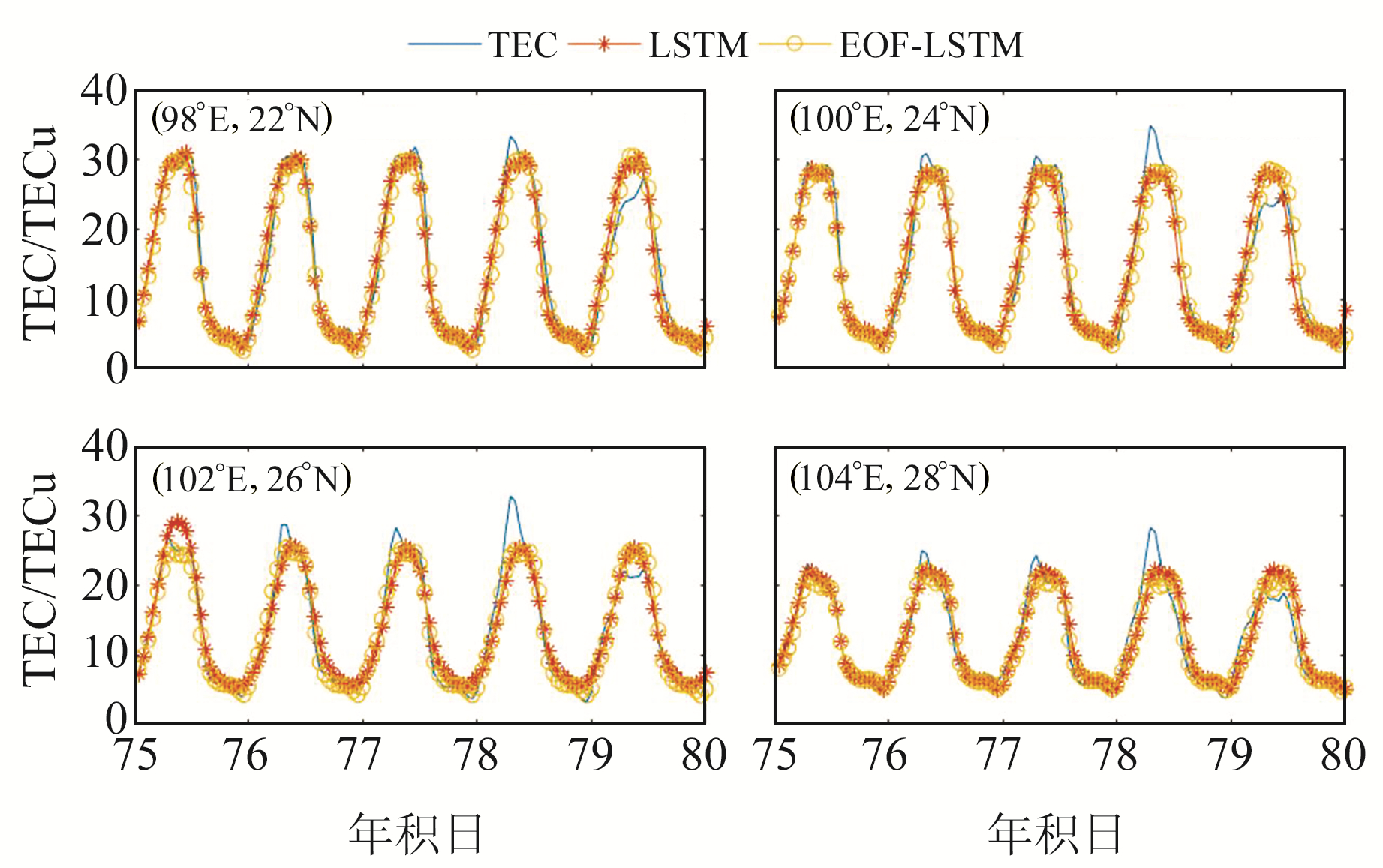图 2 不同格网点预报效果 Fig. 2 Schematic diagram of forecast effect of different grid points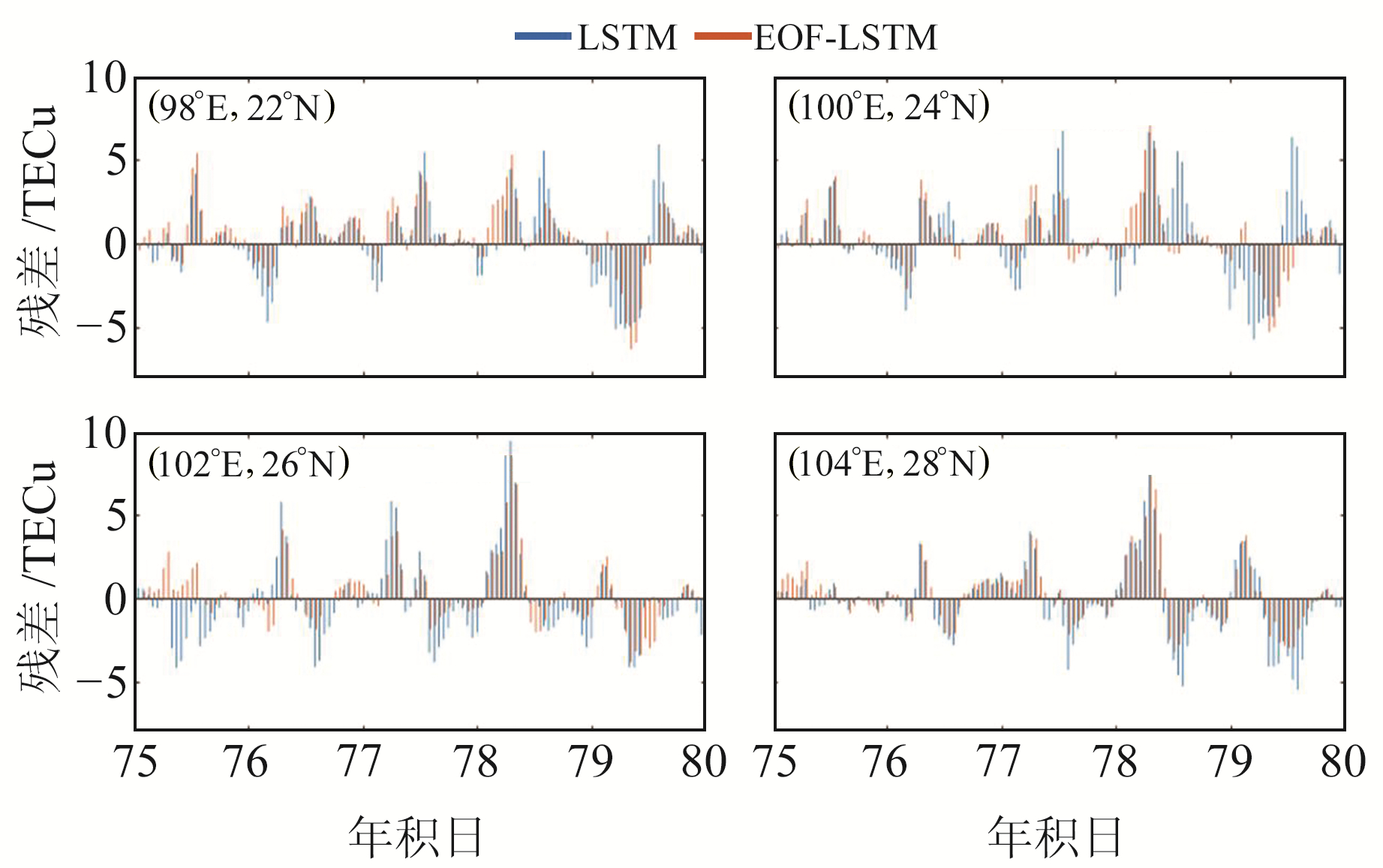图 3 不同格网点TEC残差分布 Fig. 3 TEC residual distribution of different grid points表 1 不同经纬度地区2种预报模型的评价指标 Tab. 1 Evaluation indexes of two forecasting models in different latitude and longitude areas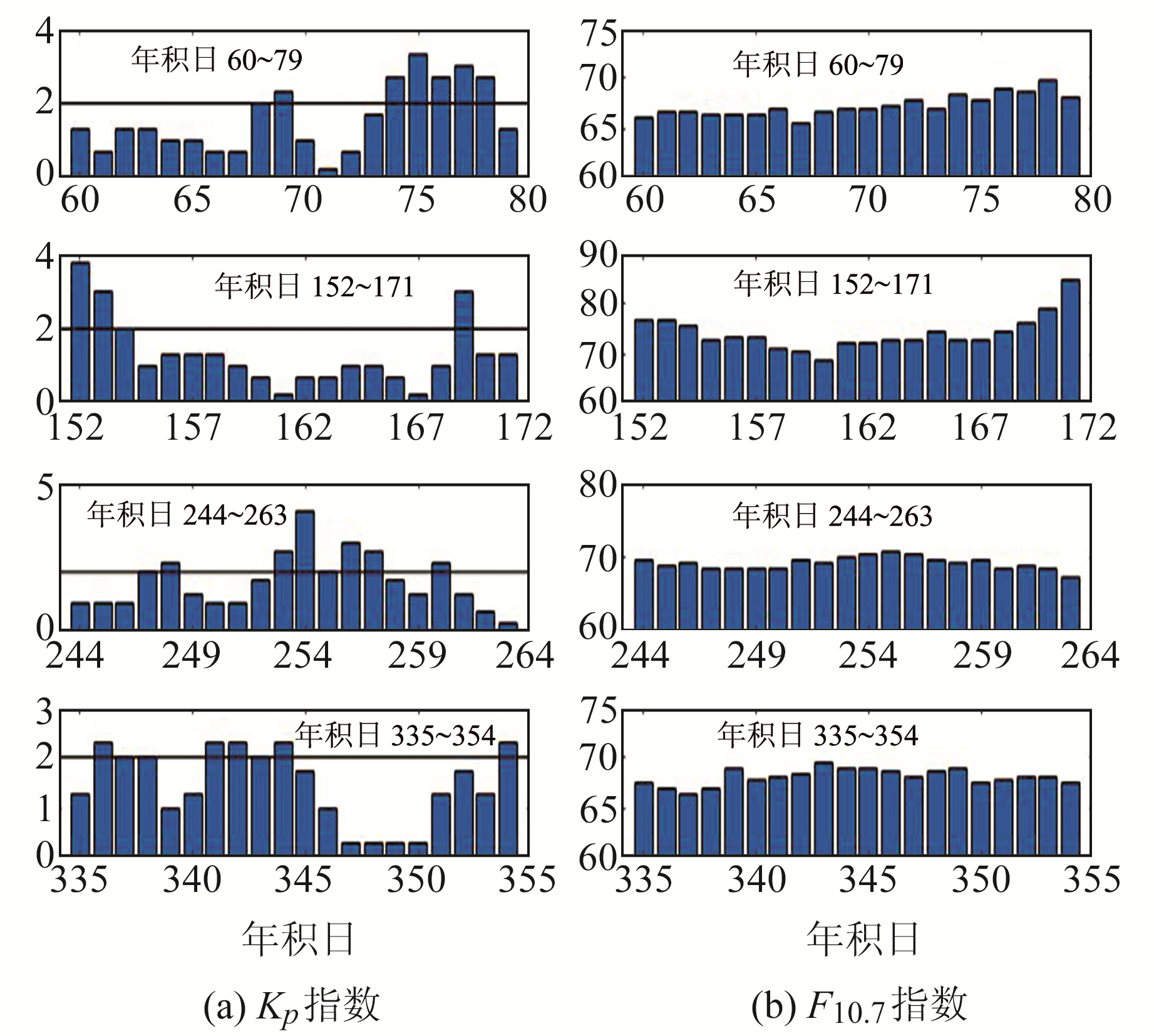图 4 不同时段的Kp指数和F10.7指数 Fig. 4 Kp index and F10.7 index in different time periods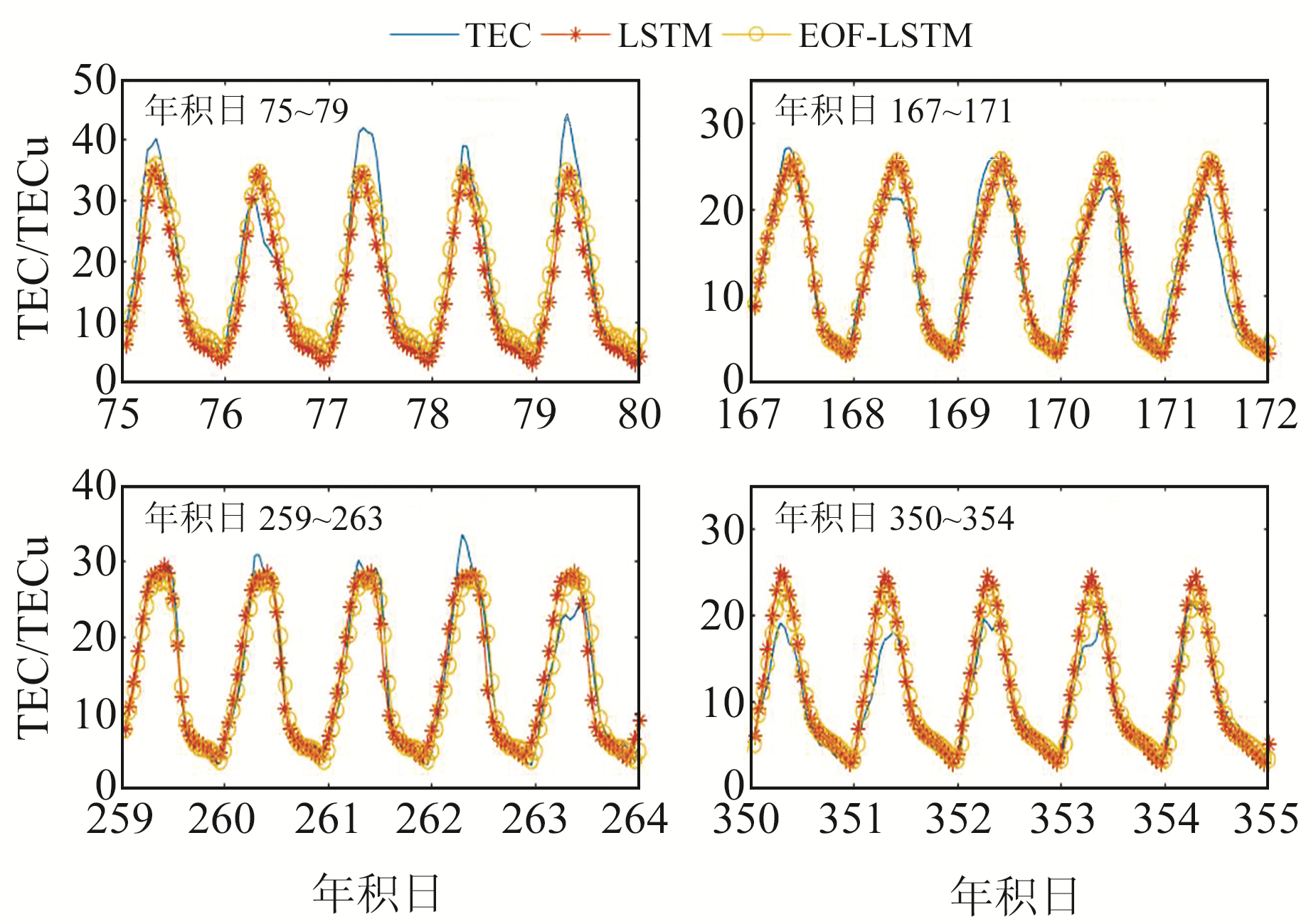图 5 (104°E，24°N)观测点预报效果 Fig. 5 Schematic diagram of forecast effect at observation point (104°E, 24°N)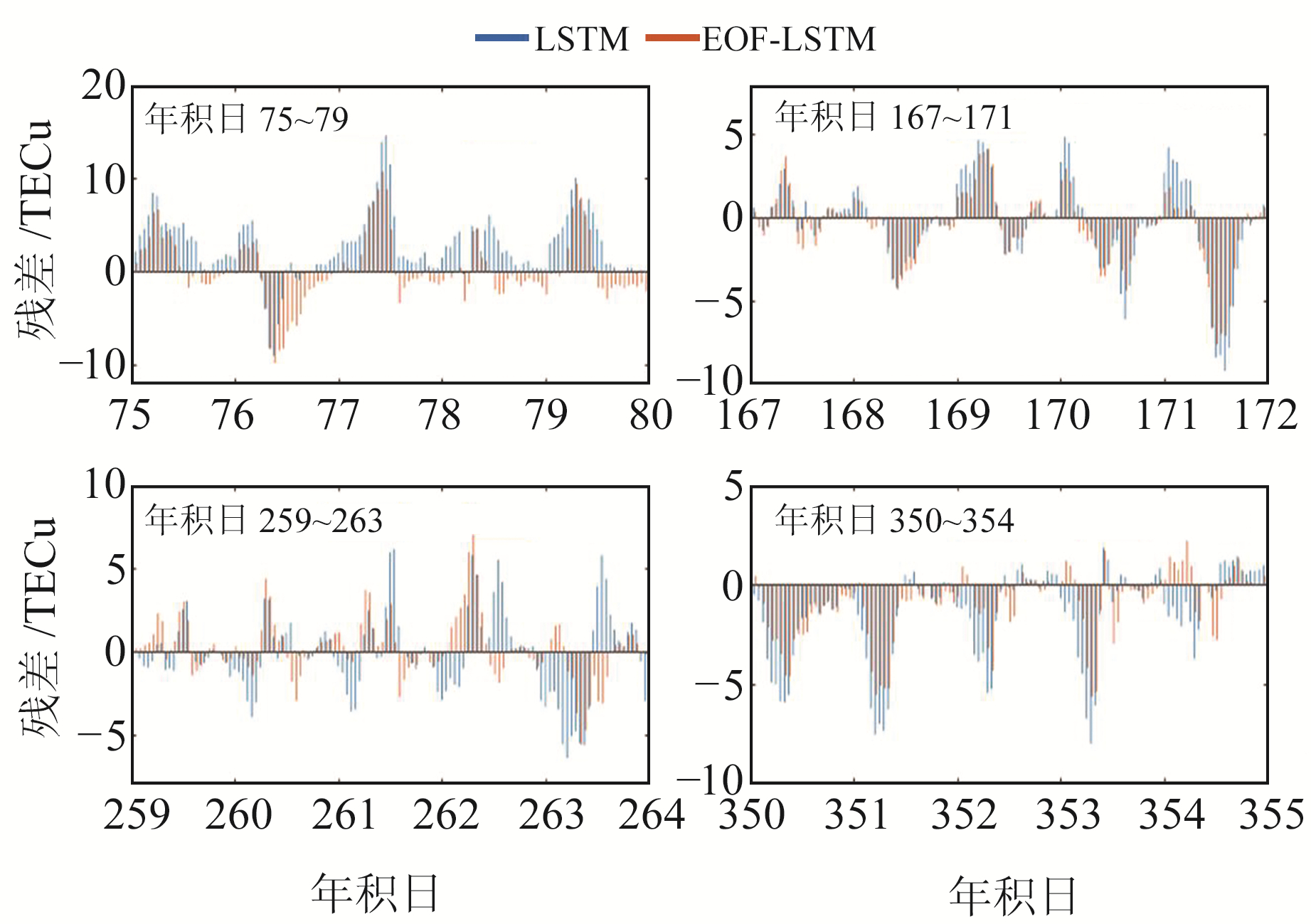图 6 (104°E，24°N)观测点不同时段TEC残差分布 Fig. 6 TEC residual distribution diagram in different time periods at observation point (104°E, 24°N)表 2 不同时段2种预报模型的评价指标 Tab. 2 Evaluation indexes of two forecasting models in different time periods表 3 不同时段残差百分比统计 Tab. 3 Statistical table of residual percentage in different time periods

3 结语

1) 首先利用EOF分解将TEC格网数据矩阵分解为空间特征和时间系数，剔除时间系数冗余信息，只针对主要时间向量进行预测重构，提高了计算效率并可更好地把握TEC序列的时间性。

2) 通过对同一时段不同地点及同一地点不同时段的TEC值进行预报分析发现，EOF-LSTM预报模型在不同经纬度、不同地磁活动和不同太阳活动条件下的预测结果均能体现出TEC的变化趋势，精度和稳定性均优于单一LSTM神经网络模型。

  李启航, 王剑, 刘瑞华. 45°(N)纬度带的Klobuchar-Like电离层延迟季节修正模型与评估[J]. 中国空间科学技术, 2019, 39(6): 30-37 (Li Qihang, Wang Jian, Liu Ruihua. A Modified Klobuchar-Like Model of Ionosphere Delay with Consideration for Seasons for 45°(N) Latitude Belt[J]. Chinese Space Science and Technology, 2019, 39(6): 30-37) (0)  程胡华, 詹彩菊, 赵亮, 等. IRI2016参考电离层模型在高度60~100 km的精度分析[J]. 测绘学报, 2020, 49(1): 42-54 (Cheng Huhua, Zhan Caiju, Zhao Liang, et al. Accuracy Analysis of IRI2016 International Reference Ionosphere at Altitude of 60-100 km[J]. Acta Geodaetica et Cartographica Sinica, 2020, 49(1): 42-54) (0)  Inyurt S, Kashani M H, Sekertekin A. Ionospheric TEC Forecasting Using Gaussian Process Regression(GPR) and Multiple Linear Regression(MLR) in Turkey[J]. Astrophysics and Space Science: An International Journal of Astronomy, Astrophysics and Space Science, 2020, 365(6): 1-17 DOI:10.1007/s10509-020-03817-2 (0)  Shi H L, Lan G W. A Grey Model for Short-Term Prediction of the Ionospheric TEC[C]. International Conference on Geomatics in the Big Data Era, Guilin, 2019 (0)  毛文军, 常文龙. 基于灰色组合模型的电离层电子含量预报[J]. 测绘科学, 2016, 41(1): 39-43 (Mao Wenjun, Chang Wenlong. Prediction of Ionospheric VTEC Using Grey Time Series Combination Model[J]. Science of Surveying and Mapping, 2016, 41(1): 39-43) (0)  张小红, 任晓东, 吴风波, 等. 自回归移动平均模型的电离层总电子含量短期预报[J]. 测绘学报, 2014, 43(2): 118-124 (Zhang Xiaohong, Ren Xiaodong, Wu Fengbo, et al. Short-Term TEC Prediction of Ionosphere Based on ARIMA Model[J]. Acta Geodaetica et Cartographica Sinica, 2014, 43(2): 118-124) (0)  Syed S, Gampala S, Madhav B T P, et al. Implementation of VARMA Model for Ionospheric TEC Forecast over an Indian GNSS Station[C]. 5th International Conference on Devices, Circuits and Systems(ICDCS), Coimbatore, 2020 (0)  谢劭峰, 陈军, 黄良珂, 等. 基于Holt-Winters的电离层总电子含量预报[J]. 大地测量与地球动力学, 2017, 37(1): 72-76 (Xie Shaofeng, Chen Jun, Huang Liangke, et al. Ionospheric TEC Prediction Based on Holt-Winters Models[J]. Journal of Geodesy and Geodynamics, 2017, 37(1): 72-76) (0)  陈雨田, 刘立龙, 刘中流, 等. 纬度因素对季节性时序TEC短期预报模型的影响分析[J]. 大地测量与地球动力学, 2018, 38(6): 581-586 (Chen Yutian, Liu Lilong, Liu Zhongliu, et al. Analysis of the Influence of Latitude Factors on Seasonal Time Series TEC Short-Term Forecast Model[J]. Journal of Geodesy and Geodynamics, 2018, 38(6): 581-586) (0)  刘淑琼, 高鑫, 李长春. EEMD-RBF神经网络的电离层TEC预报模型[J]. 测绘工程, 2020, 29(3): 15-19 (Liu Shuqiong, Gao Xin, Li Changchun. Prediction Models of Ionospheric TEC by EEMD and Radial Basis Function Neural Network[J]. Engineering of Surveying and Mapping, 2020, 29(3): 15-19) (0)  袁天娇, 陈艳红, 刘四清, 等. 基于深度学习递归神经网络的电离层总电子含量经验预报模型[J]. 空间科学学报, 2018, 38(1): 48-57 (Yuan Tianjiao, Chen Yanhong, Liu Siqing, et al. Prediction Model for Ionospheric Total Electron Content Based on Deep Learning Recurrent Neural Network[J]. Chinese Journal of Space Science, 2018, 38(1): 48-57) (0)  李淑慧, 彭军还, 徐伟超, 等. 利用神经网络预报短期电离层TEC变化[J]. 测绘科学, 2013, 38(1): 8-9 (Li Shuhui, Peng Junhuan, Xu Weichao, et al. Short-Term Ionospheric TEC Change Prediction by Neural Network[J]. Science of Surveying and Mapping, 2013, 38(1): 8-9) (0)  Li C, Peng H, Huang L K, et al. Research on Short-Term Ionospheric Prediction Combining with EOF and ARIMA Model over Guangxi Area[C]. International Conference on Geomatics in the Big Data Era(ICGBD), Guilin, 2020 (0)  苏育嵩. 经验正交分解法的基本原理及其在场预报中的应用[J]. 山东海洋学院学报, 1979(2): 50-62 (Su Yusong. The Basic Principle of Empirical Orthogonal Decomposition Method and Its Application in Field Prediction[J]. Periodical of Ocean University of China, 1979(2): 50-62) (0)  吉长东, 王强, 王贵朋, 等. 深度学习LSTM模型的电离层总电子含量预报[J]. 导航定位学报, 2019, 7(3): 76-81 (Ji Changdong, Wang Qiang, Wang Guipeng, et al. TEC Prediction of Ionosphere Based on Deep Learning LSTM Model[J]. Journal of Navigation and Positioning, 2019, 7(3): 76-81) (0)
Prediction Model of Ionospheric TEC by EOF and LSTM Neural Network
TANG Jun1,2LI Yinjian1,2     ZHONG Zhengyu1,2     GAO Xin1,2
1. School of Civil Engineering and Architecture, East China Jiaotong University, 808 East-Shuanggang Street, Nanchang 330013, China;
2. National Experimental Teaching Demonstration Center of Civil Engineering, East China Jiaotong University, 808 East-Shuanggang Street, Nanchang 330013, China
Abstract: In order to effectively utilize the time information of the ionospheric total electron content (TEC) series, we propose a prediction model combining the empirical orthogonal function decomposition and the neural network of long and short memory. We use the TEC grid data of Yunnan region provided by IGS to model and forecast the ionosphere at different locations and different periods. The experimental results of the prediction model in this paper show that the optimal root mean square error of TEC values for 5 days in the same period of time is 1.83 TECu, which is reduced by 16% compared with the single model, and the optimal average relative accuracy is 91.56%, which is increased by 7% compared with the single model. The optimal root mean square error of TEC values at the same location for 5 days is 1.86 TECu, which is 25% less than that of the single model, and the optimal average relative accuracy is 90.74%, which is 7% more than that of the single model.
Key words: empirical orthogonal function; LSTM neural network; TEC; prediction model